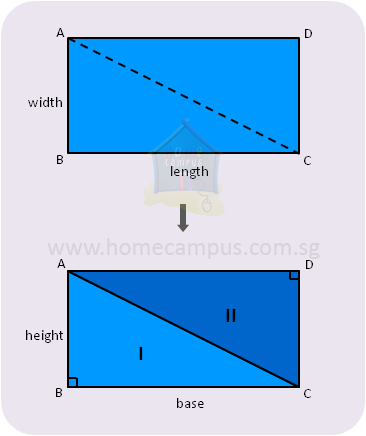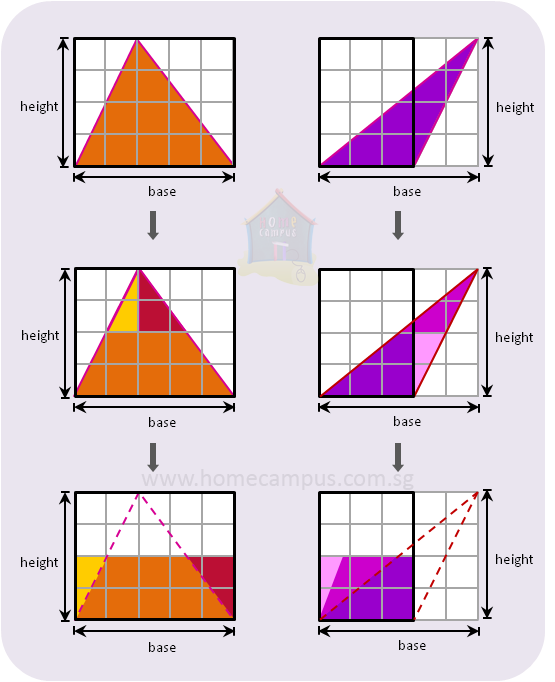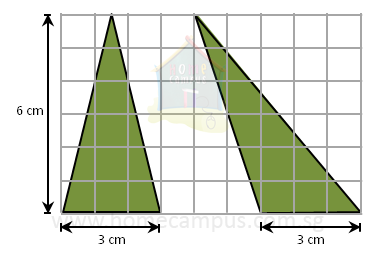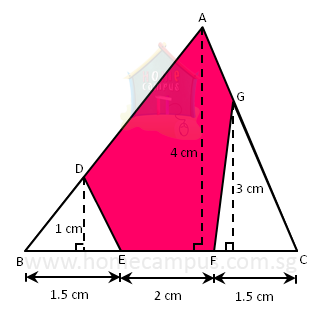## Finding the Area of a Triangle

Practice Unlimited Questions

#### Formula for Area of a Triangle

The area of a triangle  =
 1 2
×  base  ×  height

#### 1. Deriving the formula to calculate area of a triangle.

In the figure below,
Area of the rectangle ABCD  =  length  ×  width

Cut the rectangle into 2.
Now, each half is a right-angled triangle.
And, each right-angled triangle, therefore, has half the area of the rectangle.Area of right-angled triangle
 1 2
area of rectangle
 1 2
×  (length  ×  width)
 1 2
×  (base  ×  height)
Study the following examples.In both examples above, the area of the triangle is half the area of the rectangle made by the base and height.

So,
Area of a triangle
 1 2
of area of rectangle made by the base and height
 1 2
×  (base  ×  height)

#### 2. Find the area of a triangle that has a base of 3 cm and a height of 6 cm.Both triangles above have a base of 3 cm and a height of 6 cm.
Therefore, they both have the same area which is calculated as below:

Area of triangle
 1 2
×  (base  ×  height)
 1 2
×  3 cm  ×  6 cm
=   9 cm2

#### 3. Find the area of the shaded pentagon below.Area of triangle ABC
 1 2
×  (1.5 cm  +  2 cm  +  1.5 cm)  ×  4 cm
 1 2
×  5 cm  ×  4 cm
=   10 cm2

Area of triangle BED
 1 2
×  1.5 cm  ×  1 cm
=   0.75 cm2

Area of triangle GFC
 1 2
×  1.5 cm  ×  3 cm
=   2.25 cm2